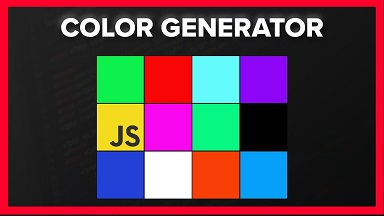# How to Generate Random Color in JavaScript

Sometimes you may need to pick a random color for your web pages or DOM elements on it. You can easily do this using plain JavaScript, although there are many third-party libraries also available for it. In this article, we will learn how to generate random color in JavaScript.

## How to Generate Random Color in JavaScript

Here are the steps to generate random color in JavaScript. We will be generating a hex color code which starts with # and is followed by 6 hex characters (0-9 & A-F). For example, #A032B1 is a hex color code.

First we will define a character set variable to contain all hex characters.

`var charset = '0123456789ABCDEF';`

Next, we initiate a string to store our hex color code.

`var color = '#';`

We will use Math.random() function to pick a hex character from our charset variable, using a random index. It returns a random number between 0 and 1 (excluding 1). We will multiply it with 16 and round it down to nearest integer to get an index between 0 and 16 (excluding 16). Here is the formula to pick random hex character.

`charset[Math.floor(Math.random() * 16)];`

Let us look at the above formula in detail. Math.floor(Math.random()*16) will return a random number between 0 and 16 (excluding 16). This is nothing but a random index we will use to pick a random character from charset string variable. We will do this 6 times since we need 6 hex characters for generating color code.

```for (var i = 0; i < 6; i++) {
color += charset[Math.floor(Math.random() * 16)];
}```

Putting it together in a JavaScript function,

```function getRandomColor() {
var charset = '0123456789ABCDEF';
var color = '#';
for (var i = 0; i < 6; i++) {
color += charset[Math.floor(Math.random() * 16)];
}
return color;
}```

You can use this function to use random color for CSS, as shown below.

```function setRandomColor() {
\$("#myDiv").css("background-color", getRandomColor());
}```

In this article, we have learnt how to generate random color in JavaScript.